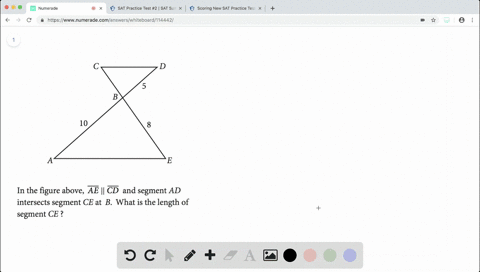Enroll in one of our FREE online STEM summer camps. Space is limited so join now!View Summer Courses### In the figure above, \$\overline{A E} \| \overline…

02:31Johns Hopkins University

Need more help? Fill out this quick form to get professional live tutoring.

Get live tutoring
Problem 17

Which of the following expresses the riser height in
$$\begin{array}{l}{\text { A) } h=\frac{1}{2}(25+d)} \\ {\text { B) } h=\frac{1}{2}(25-d)} \\ {\text { C) } h=-\frac{1}{2}(25+d)} \\ {\text { D) } h=-\frac{1}{2}(25-d)}\end{array}$$

Check back soon!
SAT
SAT Practice Test # 6

## Discussion

You must be signed in to discuss.

## Video Transcript

So for this one it says that we want to express the riser height in terms of the tread death. We want to express something in terms of the other things. That means two isolate whatever its has to express. So we want to isolate each well. We have the equation right here to age. Post E is equal to twenty five. So we just have to take that. And I say that h In order to do so, I'm going to subtract D from both sides. Minutes to age is twenty five months D. From here on this one divide both sides by two, which is going to give us a TSH is equal to one half, twenty five minutes. Game to that is going to be. It's like B.